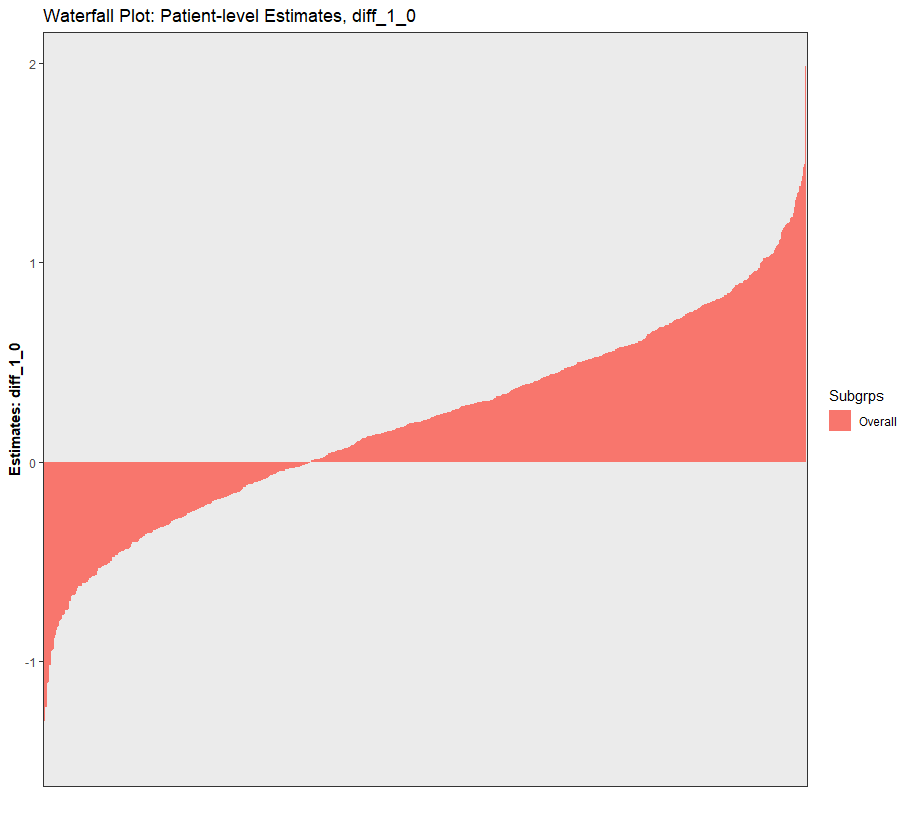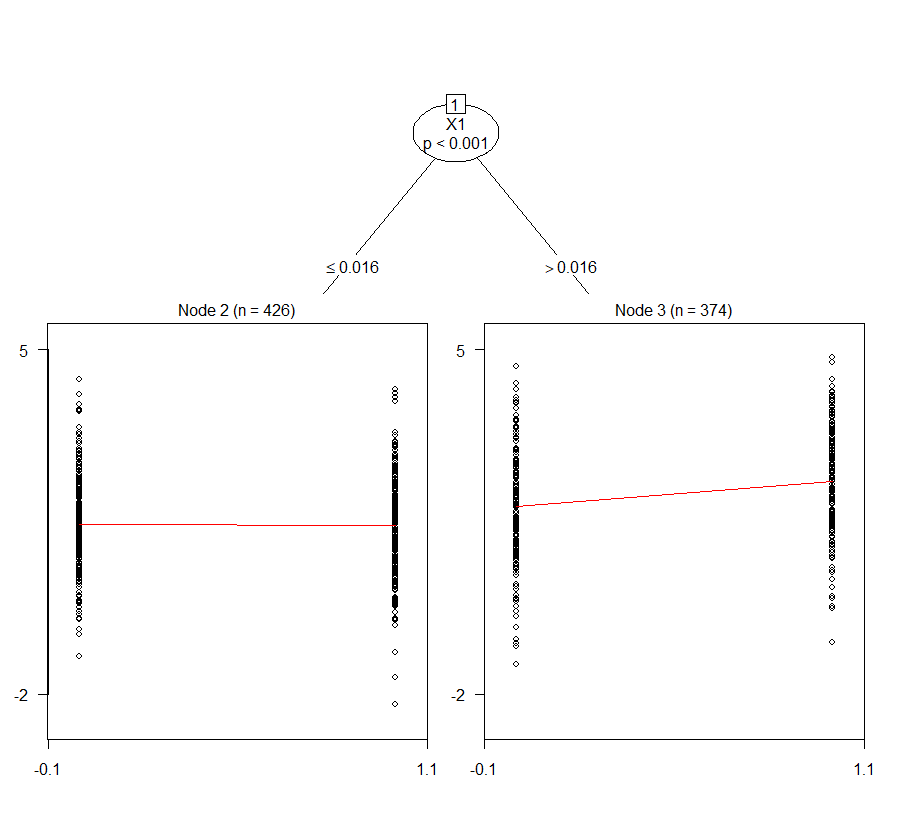# User-specific Models: StratifiedMedicine

One advantage of the “StratifiedMedicine”" package is the flexibility to input user-created functions/models. This facilitates faster testing and experimentation. First, let’s simulate the continuous data again.

library(StratifiedMedicine)
dat_ctns = generate_subgrp_data(family="gaussian")
Y = dat_ctns$Y X = dat_ctns$X # 50 covariates, 46 are noise variables, X1 and X2 are truly predictive
SE = summary(rlm.mod)$coefficients[2,2] LCL = est-qt(1-alpha/2, n-1)*SE UCL = est+qt(1-alpha/2, n-1)*SE pval = 2*pt(-abs(est/SE), df=n-1) param.dat <- data.frame(N= n, estimand = "mu_1-mu_0", est=est, SE=SE, LCL=LCL, UCL=UCL, pval=pval) return(param.dat) } # Putting it All Together Finally, let’s input these user-specific functions into each step along with combining the components with PRISM: step1 <- filter_train(Y, A, X, filter="filter_lasso") X.star <- X[,colnames(X) %in% step1$filter.vars]
step2 <- ple_train(Y, A, X.star, ple = "ple_ranger_mtry")
plot_ple(step2)step3 <- submod_train(Y, A, X.star, submod = "submod_lmtree_pred")
plot(step3$fit$mod)step4 <- param_est(Y, A, X.star, Subgrps=step3$Subgrps.train, param="param_rlm") step4 #> Subgrps N estimand est SE LCL UCL #> 1 ovrl 800 mu_1-mu_0 0.234728163 0.07708495 0.08341524 0.3860411 #> 2 2 426 mu_1-mu_0 -0.004165125 0.10352507 -0.20765001 0.1993198 #> 3 3 374 mu_1-mu_0 0.506836561 0.11525208 0.28021130 0.7334618 #> pval alpha #> 1 2.402725e-03 0.05 #> 2 9.679263e-01 0.05 #> 3 1.429483e-05 0.05 # PRISM # res_user1 = PRISM(Y=Y, A=A, X=X, family="gaussian", filter="filter_lasso", ple = "ple_ranger_mtry", submod = "submod_lmtree_pred", param="param_rlm") plot_ple(res_user1)# variables that remain after filtering # res_user1$filter.vars
#>   "X1"  "X2"  "X3"  "X5"  "X7"  "X8"  "X10" "X12" "X16" "X18" "X24" "X26"
#>  "X31" "X40" "X46" "X50"
# Parameter estimates/inference
res_user1\$param.dat
#>   Subgrps   N  estimand          est         SE         LCL       UCL
#> 2       2 426 mu_1-mu_0 -0.004165125 0.10352507 -0.20765001 0.1993198
#> 3       3 374 mu_1-mu_0  0.506836561 0.11525208  0.28021130 0.7334618
#> 1    ovrl 800 mu_1-mu_0  0.234728163 0.07708495  0.08341524 0.3860411
#>           pval alpha  Prob(>0)
#> 2 9.679263e-01  0.05 0.4839537
#> 3 1.429483e-05  0.05 0.9999945
#> 1 2.402725e-03  0.05 0.9988368

# Conclusion

Overall, key tools of “StratifiedMedicine” are customizable, allowing for fast experimentation and improvement of individual steps. The main consideration for customizing the steps are certain required inputs/outputs.